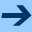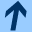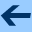Manual

# Manual

## Input Language

### Global declarations

 ::= + ::= eps  | channel (",")* ";" ::= eps  | var + ::= (",")* ":" ";" ::= bool | uint "(" ")" | sint "(" ")" ::= system (",")* ";" ::= control (",")* ";" ::= "#"  |   | "." ::= initial ";" ::= final ";"

The <channel decl> declaration defines the set of synchronization channels that can be used by processes. Processes synchronizes on channel names with a CSP-like semantic (i.e., n-ary synchronization). The <variable decl> declaration defines the set of global variables of the system. Global variables may be read by any process, and may be assigned during a process transition only if the transition is no synchronized on a channel1. Global variables can be initialzied only by some process (no global <init> declaration).

The <process> declarations defines a set of processes (see next paragraph). The <system> declaration defines which processes are considered for building the global system. The <control> declaration defines the initial partition used to perform verification. <#><name> tells that the partition should enumerate the locations of process <name>, <name> (resp. <name>"."<name>) that the partition should enumerate the values of global variable <name> (resp. local variable <name>"."<name>).

In a <type> declaration, uint and sint respectively define an unsigned integer and a signed integer represented with <integer> bits.

The <initial> and <final> declarations defines initial and final conditions.

### Process definition

 ::= process "{" + "}" ::= eps  | sync (",")* ";" ::= init ";" ::= loc ":" * ::= when goto ";" ::= eps  | sync ::= | "{" "}" ::= ::= ";" | ::= ::= ::= eps  | assign "{" "}" ::= ";" | ";" | ::= ":="
<sync decl> declaration defines the set of channels on which the

process should synchronize. <variable decl> defines a set of local variables. Local variables can be freely assigned by the owner process and read by any process. <init> declaration defines the set of initial states of the process.

A location is defined by its name and its set of outgoing nails. Transitions are guarded by Boolean expressions, can optionaly be synchronized on a channel. The destination is specified either by a single destination location, or by a distribution on destination locations. To each destination location can be associated an action, which is a set of assignmements on local variables, and also global variables if there the transition doesn't involve synchronization.

### Expressions

 ::= | | | | | if then else | "#" + | "(" ")" :: true  | false  |  :: + ::=  |  "." ::= "#"  | "#" "." ::= "not"  | "-" ::= "=>"  | "or"  | "and"  | "<=>"  | "xor" | "<>"  | "="  | ">="  | ">" | "<=" | "<" | "+"  | "-"  | "*"

The operators have the usual meaning. "#()" is the exclusive operator from the LUSTRE language, which returns true iff at most one of its operand is true. Their priorities are the following ones:
"-" > "*" > { "+","-" } >
{ "<>", "=", ">=",">","<=","<" } >
{ "not", "#()" } > { "<=>","xor" } > "and" > "or" > "imply" > "if then else"
The atoms of expressions are either constants (Boolean or integers one), either reference to a variable, either reference to a location. <var> refers to a local variable of the enclosing process if it exists, or otherwise the global variable. <name>"." <procname>"."<varname> refers to the local variable of another process. Last, "#"<locname> (resp. "#"<procname>"."<locname>) is a Boolean expression that is true if the enclosing process (resp. the process <procname>) is currently is the location <locname>.

## Version & Options of the tool

### Three versions

 Name LP solver Characteristics probd.opt CDDLIB dense matrices and usual floating point arithmetic probr.opt CDDLIB dense matrices and exact rational arithmetic probf.opt CDDLIB dense matrices and multiprecision floating point arithmetic probsd.opt LP_SOLVE sparse matrices and usual floating point arithmetic probsld.opt LP_SOLVE sparse matrices and long double floating point arithmetic

### Short description

```-bmaxmem <n>  : sets the max. number of MB usable by the bdds, default is 64
-gbdd <n>     : sets the ratio used/max to 1/2^n, default is 18, try up to 20
-bdd (+/-)(a|r): bdd options, default -a-r
a: enables automatic variable reordering
r: enables variable reordering
-goal (i|s|d)<fl>: goal; try to prove:
i: pinf >= fl
s: psup <= fl
d: (psup-pinf) <= fl (the default, with fl=0.0)
-epsilon <n>  : consider value v<epsilon as zero, default is 1e-30
-precision <n>: use <n> bits at most in GMP float numbers, default is 50
-enum (+/-)(a|r): analysis options, default +a+r
a: uses enumerative method for Boolean analysis
(always the case with essential automaton)
r: uses enumerative method for refinement
-ess (+/-)(a|r): essential states options, default +a+r
a: uses essential locations for analysis
r: refines only essential locations
-bindiv str: uses binary divisions in refinement (the default)
str should be 'b' or any permutation of 'lso',
gives the priority of types of nails in refinement
l: looping nail
s: single successor nails
o: other nails
b: choose the nail in order to balance (nb of states divided by two)
-narydiv str: uses nary divisions in refinement (the default)
str should be 'a' or any permutation of 'lso',
gives priority of nails ccording to which refinement is performed
l: looping nail
s: single successor nails
o: other nails
a: all nails
-ratio <n>    : ratio in percentage between new and old size, before new
analysis.
n > 100, default is 150.
-bdd_limit <float>: maximum nb of minterms in DDs that allows display
-debug <n>    : debug mode, 0 <= n <= 2
-verbose <n>  : verbose mode, 0 <= n <= 2
-stat         : shows statistics at end
-help  display this list of options
```

### Hints

Autoreordering doesn't work well. Reordering (`-bdd +r`) takes some time, but later may speed up refinement operations.

You should also play with `ratio`, `-bindiv` and `-narydiv` options.

If there are many nails, priority in refinement should be given to "other" nails. Otherwise, these are duplicated and that generates a huge number of complex nails. This has been observed on the dining philosopher, even if this case, as we have to compute Pinf, one might think that we should split according to looping nails.

Bertrand Jeannet, July 18, 2006 - Updated on March 14, 2017 by Hubert Garavel and Jean-Philippe GrosManual